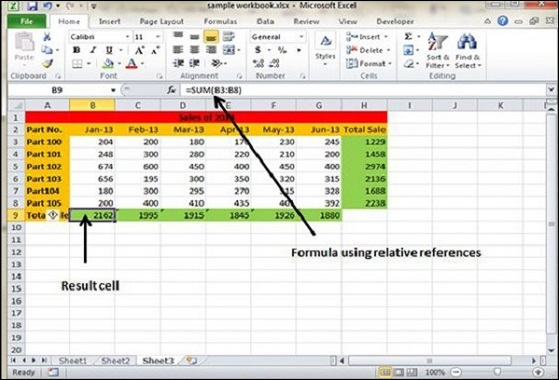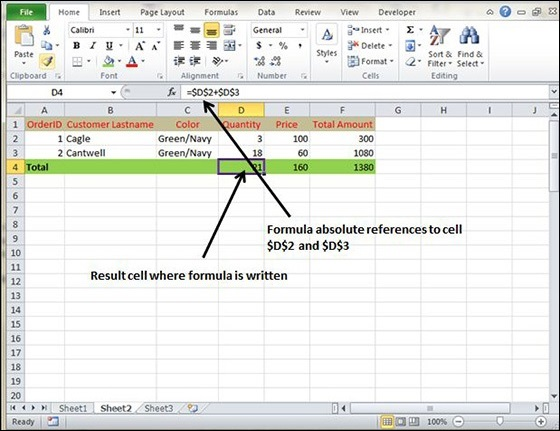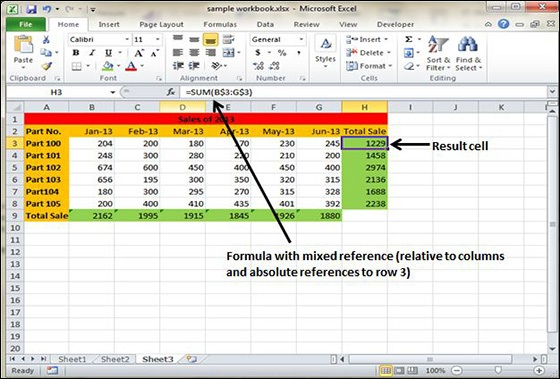Formula Reference in Excel 2010

Cell References in Formulas

Most formulas you create include references to cells or ranges. These references enable your formulas to work dynamically with the data contained in those cells or ranges. For example, if your formula refers to cell C2 and you change the value contained in C2, the formula result reflects new value automatically. If you didn’t use references in your formulas, you would need to edit the formulas themselves in order to change the values used in the formulas.

When you use a cell (or range) reference in a formula, you can use three types of references − relative, absolute, and mixed references.

Relative Cell References

The row and column references can change when you copy the formula to another cell because the references are actually offsets from the current row and column. By default, Excel creates relative cell references in formulas.Absolute Cell References

The row and column references do not change when you copy the formula because the reference is to an actual cell address. An absolute reference uses two dollar signs in its address: one for the column letter and one for the row number (for example, \$A\$5).Mixed Cell References

Both the row or column reference is relative and the other is absolute. Only one of the address parts is absolute (for example, \$A5 or A\$5).Useful Video Courses

Video

MS Excel Online Training

Most Popular

102 Lectures 10 hours

Video

Excel VBA Online Training

101 Lectures 6 hours

Video

Advanced Excel (Power Query) Online Training

Best Seller

56 Lectures 5.5 hours

Video

Excel Dashboard Crash Course

63 Lectures 3.5 hours

Video